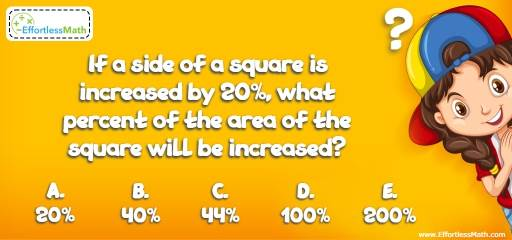# Geometry Puzzle – Challenge 65

Who can solve this math puzzle? This is a great math and critical thinking challenge to help you develop your creative thinking!## Challenge:

If a side of a square is increased by $$20\%$$, what percent of the area of the square will be increased?

A- $$20\%$$

B- $$40\%$$

C- $$44\%$$

D- $$100\%$$

E- $$200\%$$

### The Absolute Best Book to Challenge Your Smart Student!

Area of a square = side times side.
Let X be the side of a square. Then, the side of the square is $$X^2$$.
If a side of a square is increased by $$20\%$$, then the area of the square will be increased by 44 percent:
$$1.2X × 1.2X = 1.44X^2$$
$$1.44X^2$$ is 0.44 or $$44\%$$ bigger than $$X^2$$

The Best Books to Ace Algebra

### What people say about "Geometry Puzzle – Challenge 65 - Effortless Math: We Help Students Learn to LOVE Mathematics"?

No one replied yet.

X
45% OFF

Limited time only!

Save Over 45%

SAVE $40 It was$89.99 now it is \$49.99